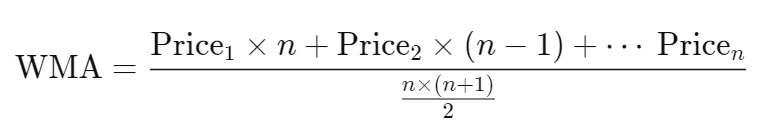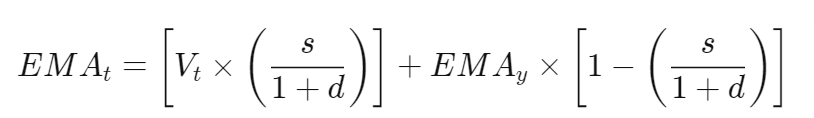# Moving Averages

In fast-moving markets, it can often be observed that the price of a stock is skyrocketing, only to plummet sometime later. These significant inconsistencies can potentially create false signals.

Moving averages help to filter out the noise from such volatile price movements and act as trend-following indicators.

## What is a Moving Average (MA)?

A moving average is a commonly used technical analysis tool used to smooth out price data and obtain an average value.

Moving averages are computed to determine a stock's trend direction or support level and resistance levels.

Primarily, when the price level of a stock rises above the moving average line, traders consider it as an indication to buy. And when the price falls below this line, traders contemplate it as a signal to sell.

## Different Types of Moving Averages

Primarily, there are three moving average types, and they are explained below-

• ### Simple Moving Average

A simple moving average or SMA can be a plot by calculating the average price of a stock over different time frames. These are mainly formed based on the closing prices.

Formula for Calculating the Simple Moving Average-This can be cited better with a moving average example.

The first requirement for calculating simple moving averages is to find out the average prices of a given period and then divide their sum by the total number of periods.

Let’s say Robin wants to calculate the simple moving average for XYZ Stock by considering the closing prices of the last 5 days.

The closing prices of the last 5 days are given by Rs. 24, Rs 25.50, Rs. 24.75, Rs 25.10 and Rs 24.60

The SMA can be computed as given below:

SMA = Rs. (24 + 25.50 + 24.75 +25.10 + 24.60) / 5

Therefore, SMA= Rs. 24.79

• ### Weighted Moving Average

A weighted moving average (WMA) counters the various drawbacks of SMA. It puts more weight on recent data instead of the past. WMA follows the different price levels of stock more strictly than SMA.

Formula for Calculating the Weighted Moving Average-• ### Exponential Moving Average

Also referred to as EMA, this involves complex calculations. Similar to WMA, EMA puts more weight on the latest prices of a financial instrument.

If a 100-day EMA and a 100-day SMA are plotted on the same chart, it can be seen that the former reacts faster than the latter. This happens due to greater emphasis on the recent prices.

Formula for Calculating the Exponential Moving Average-## Difference Between Simple Moving Average (SMA) vs Exponential Moving Average (EMA)

Given below is a tabular representation showing how exponential and weighted moving averages are different from simple moving averages-

 Point of Difference SMA EMA and WMA Response to price changes SMA is slow to respond to price changes. EMA and WMA respond faster to changing prices. Weight on recent periods It gives equal weight to all periods. These put more weight on recent periods. Emphasis on traders’ actions It doesn’t emphasise on traders’ actions. These emphasise on what the traders are doing at the moment. Ability to reflect a quick shift in market sentiment. SMAs are efficient in reflecting a quick shift in sentiment. These possess the ability to reflect shifts in market sentiment.

## Importance of Moving Average Method

The direction of a moving average line assists the trader in understanding which way the price of a financial instrument is moving.

If the price of a financial instrument is above the moving average line, it is said to be on an uptrend. On the flip side, if its price is under the moving average line, it’s on a downtrend.

If the moving average line of a stock doesn’t show any vertical movements for a long period of time, it indicates that the stock price is ranging and not trending. This is observed when a stock is traded between constant high and low prices for a certain period.

Moving averages also work as support and resistance indicators for traders. Most times, the price of stock finds support at the moving average line when the trend is up. Conversely, it meets with resistance at the line when the trend is down.

Also known as a lagging indicator, a moving average line is based on previous closing prices. Hence, instead of giving a warning beforehand, it will only confirm a change in trend.

## Merits of Moving Average Method

• Moving averages help in identifying the trends. This allows the traders to avail of and understand the trends established in the market.

• It also acts as a support system as it helps in determining potential price support.

• It provides the support to measure the momentum as well. It helps to determine the direction and strength of the asset’s momentum.

## Demerits of Moving Averages

Although calculating moving average offers a quick and easy way to identify trends of financial instruments, they have the following disadvantages:

• Since each stock or commodity has its unique price history, no set rules can be implemented across all markets. Hence, a moving average cannot show the constant changes in their prices.

• The primary purpose of identifying a trend is to predict the future values of the stock. But, if the security does not trend up or down, calculating moving averages will not be able to provide the traders with an opportunity to profit.

• Stocks often tend to show a cyclical behavioural pattern that cannot be interpreted by a moving average.

• Moving averages have the ability to be spread out over different time frames, but this can become quite tricky in specific situations.

• Similar to other technical analysis methods, moving averages do not consider the changes in primary factors that have an effect on the market price of a stock. Changes in the managerial structure of a company, changes in product demand of industry are also not taken into account.
Open a free demat account
Set it up in just 2 minutes to start investing in the stock market
EXPLORE NOW